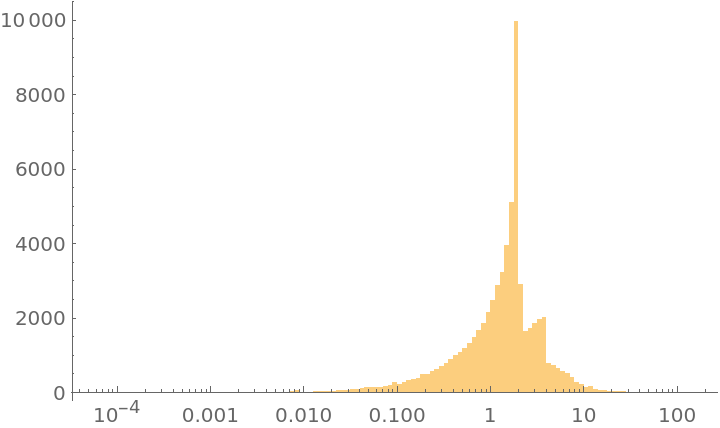#Function Repository Resource:

# Cos2PiOverFermatPrime

Closed form of cos(2π/p) where p is a Fermat prime (3, 5, 17, 257, 65537) a la Gauss

Contributed by: Michael Trott
 ResourceFunction["Cos2PiOverFermatPrime"][p,"Rules"] returns a list {cos,rules} where cos represents cos(2π/p) and rules is a list of replacements to be applied to cos. The value p can be one of the five Fermat primes 3, 5, 17, 257, 65537. ResourceFunction["Cos2PiOverFermatPrime"][p,trig] returns trig(2π/p) where trig can be any of the trigonometric function Cos,Sin,Tan,Cot,Sec, or Csc.

## Details and Options

ResourceFunction["Cos2PiOverFermatPrime"] is a faithful implementation of Gauss’ algorithm discovered on March 29 in 1796.
The original result realized a ruler and compass construction of a regular 17‐gon.
The resulting expressions contain only square roots, no cube roots or higher roots.
The option GeneratedParameters can be used to specify the variable used to express the rules.

## Examples

### Basic Examples

The classic Gauss result for cos(2π/17):

 In:=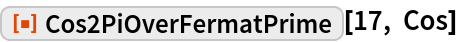Out=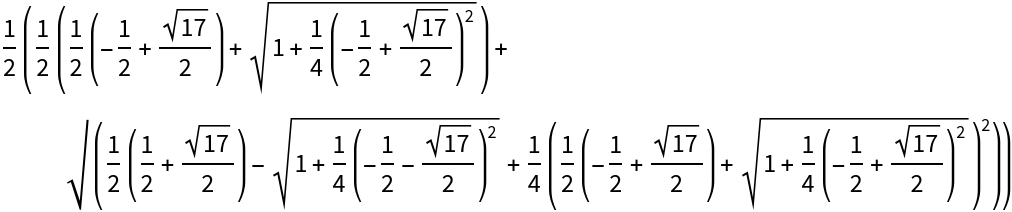Using the "Rules" argument shows the recursive nature of the period values:

 In:=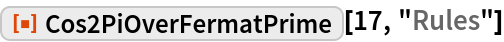Out=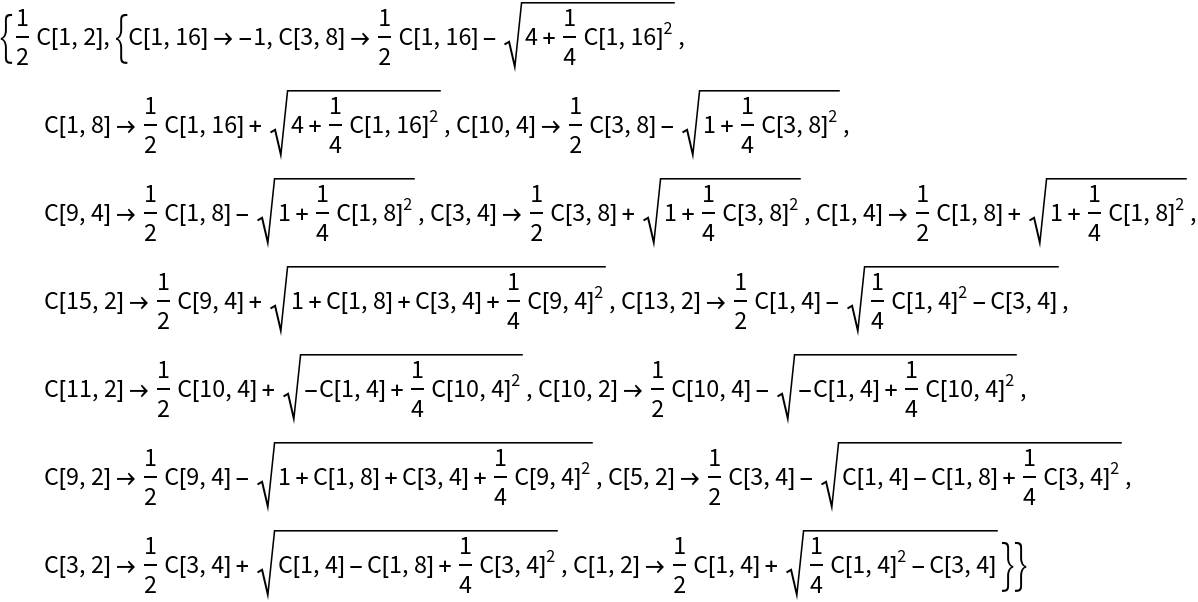Applying the rules gives again the above expression:

 In:=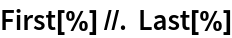Out=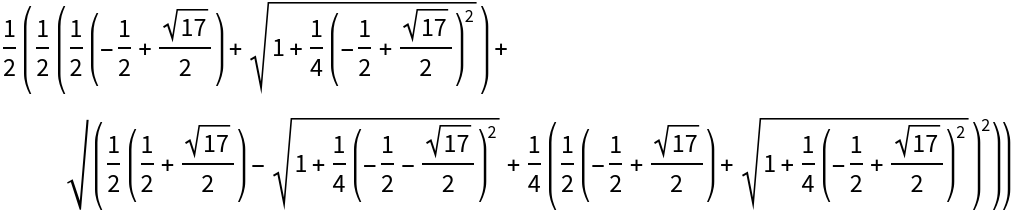The cosine values for the two smallest Fermat primes:

 In:=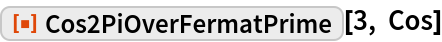Out=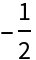In:=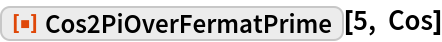Out=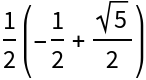### Scope

Cos2PiOverFermatPrime gives results for all the basic trig functions: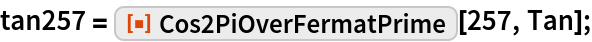In:=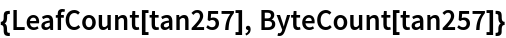Out=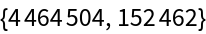In:=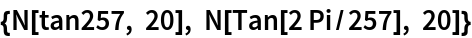Out=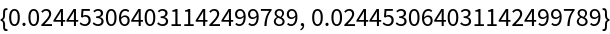### Options

The default parameter is C:

 In:=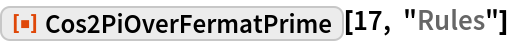Out=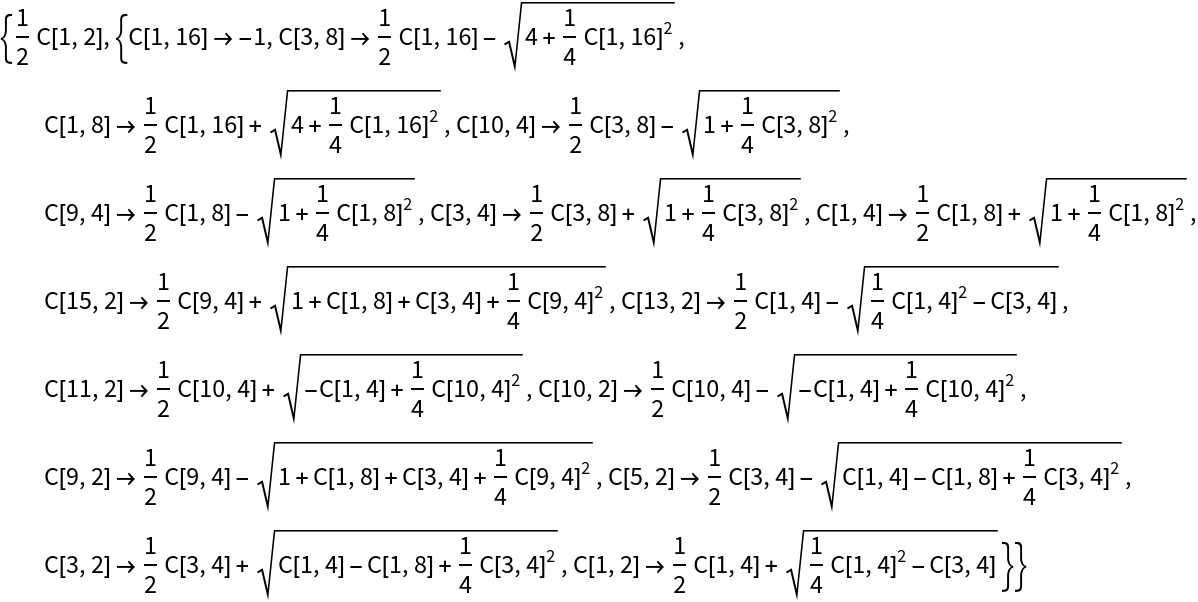Use subscripts for the period values:

 In:=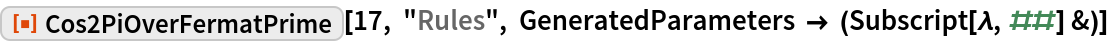Out=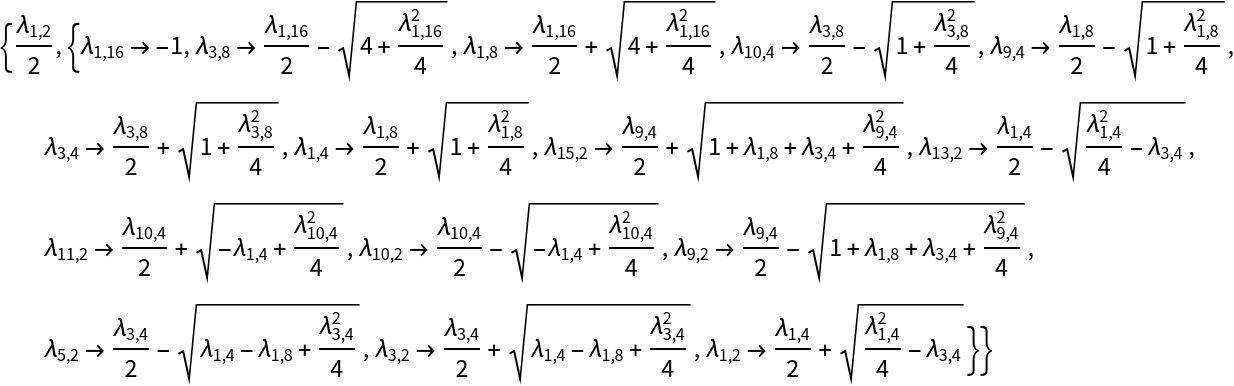### Applications

The case p=257 was manually computed by Richelot in 1832:

 In:=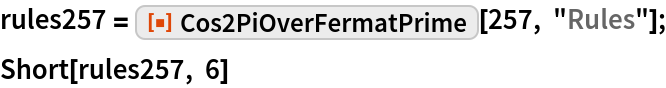Out=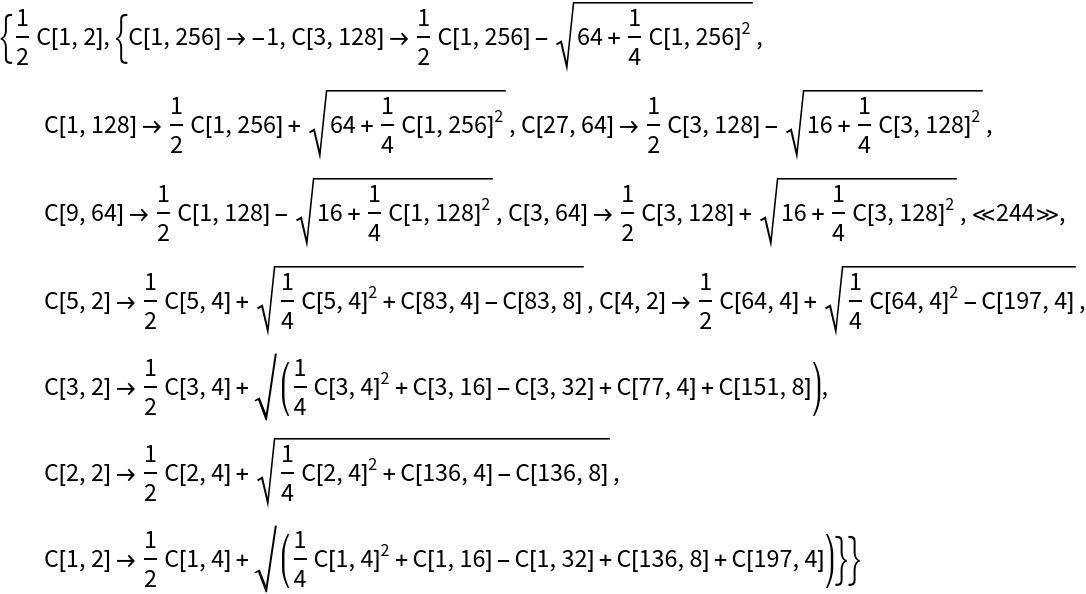In:=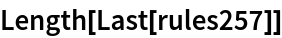Out=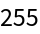cos(2π/257):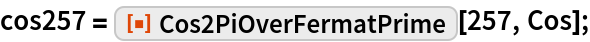The expression contains more than 5,100 square roots:

 In:=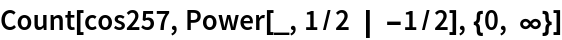Out=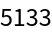Plot the frequency of occurrences of different square roots (mouseover points to see square roots):In:=Out=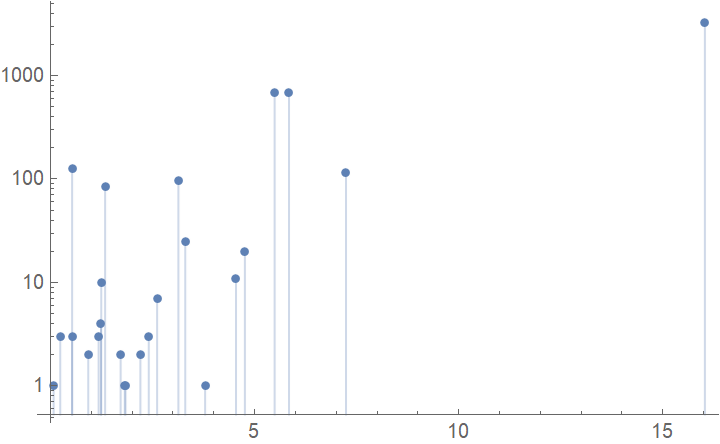The network of the period values of cos(2π/257):

 In:=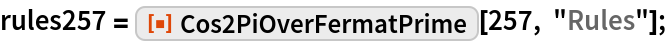Visualize with a graph:

 In:=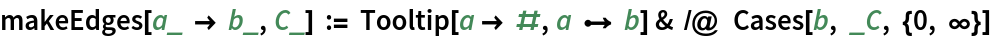In:=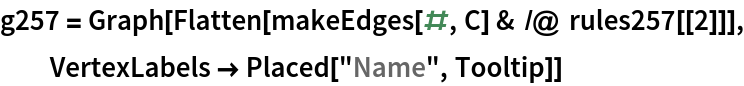Out=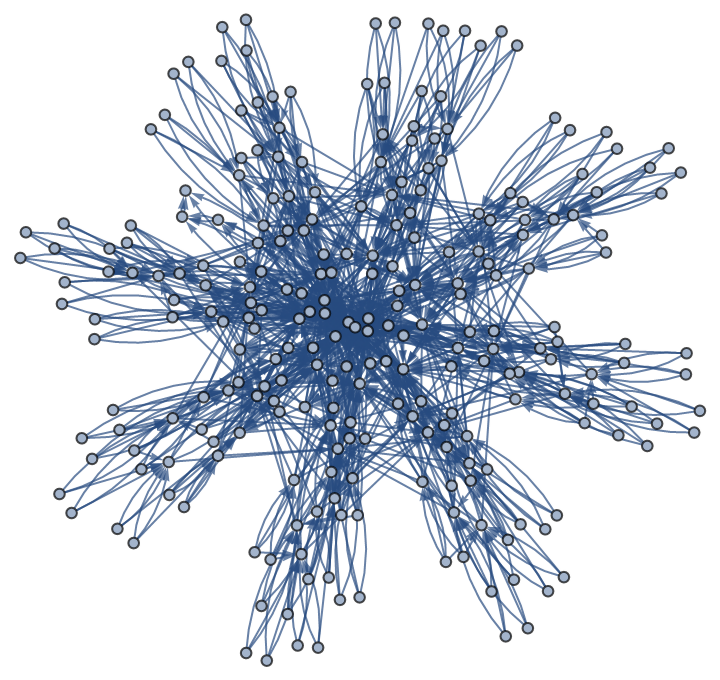In:=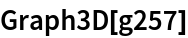Out=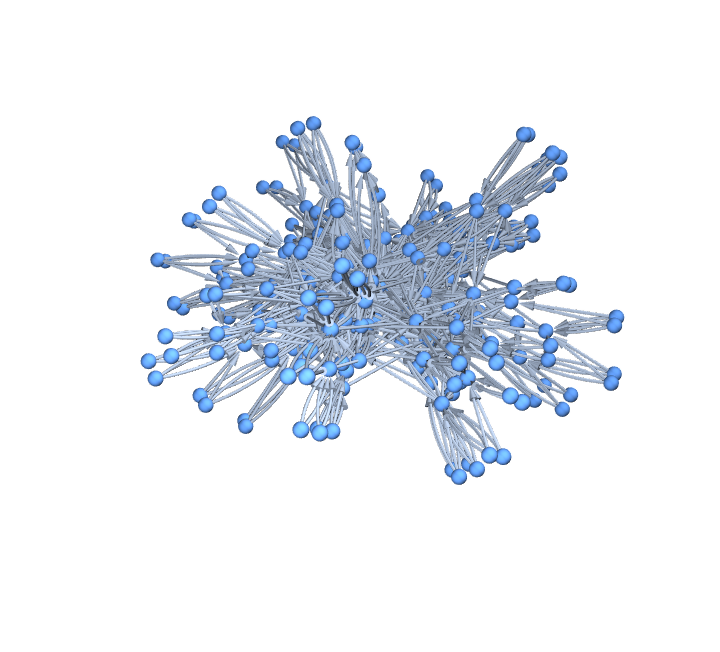Find the explicit numerical values of all periods:

 In:=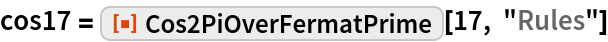Out=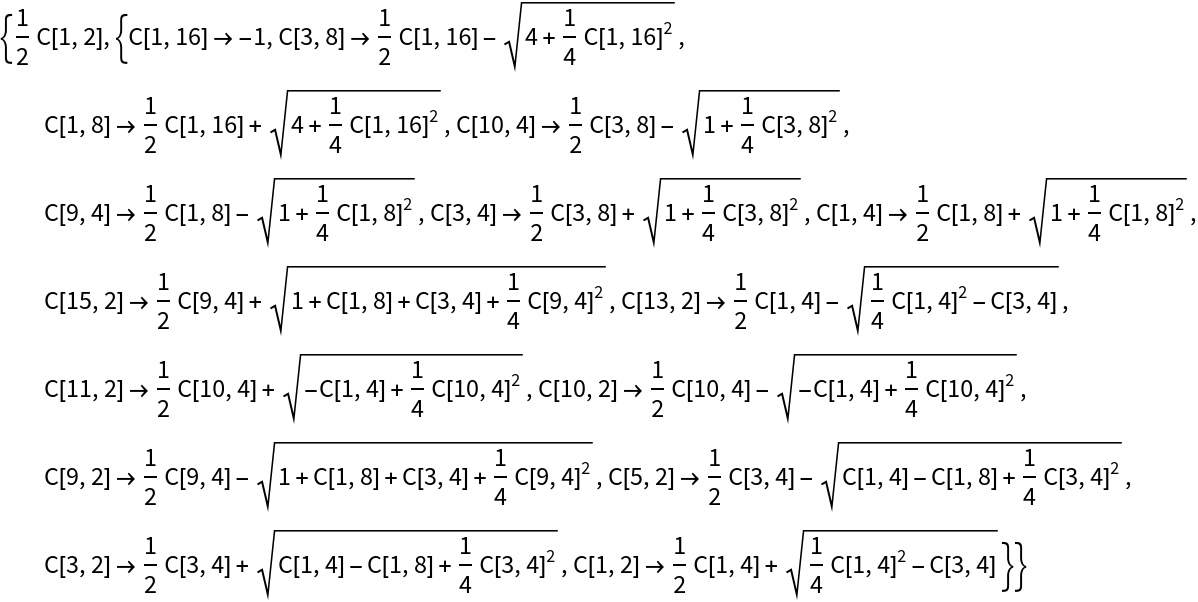In:=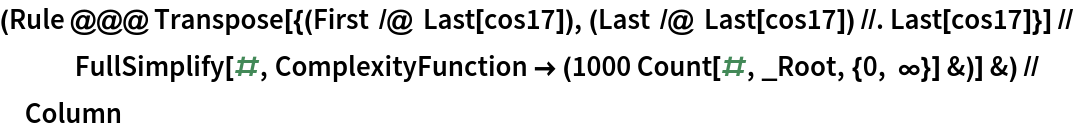Out=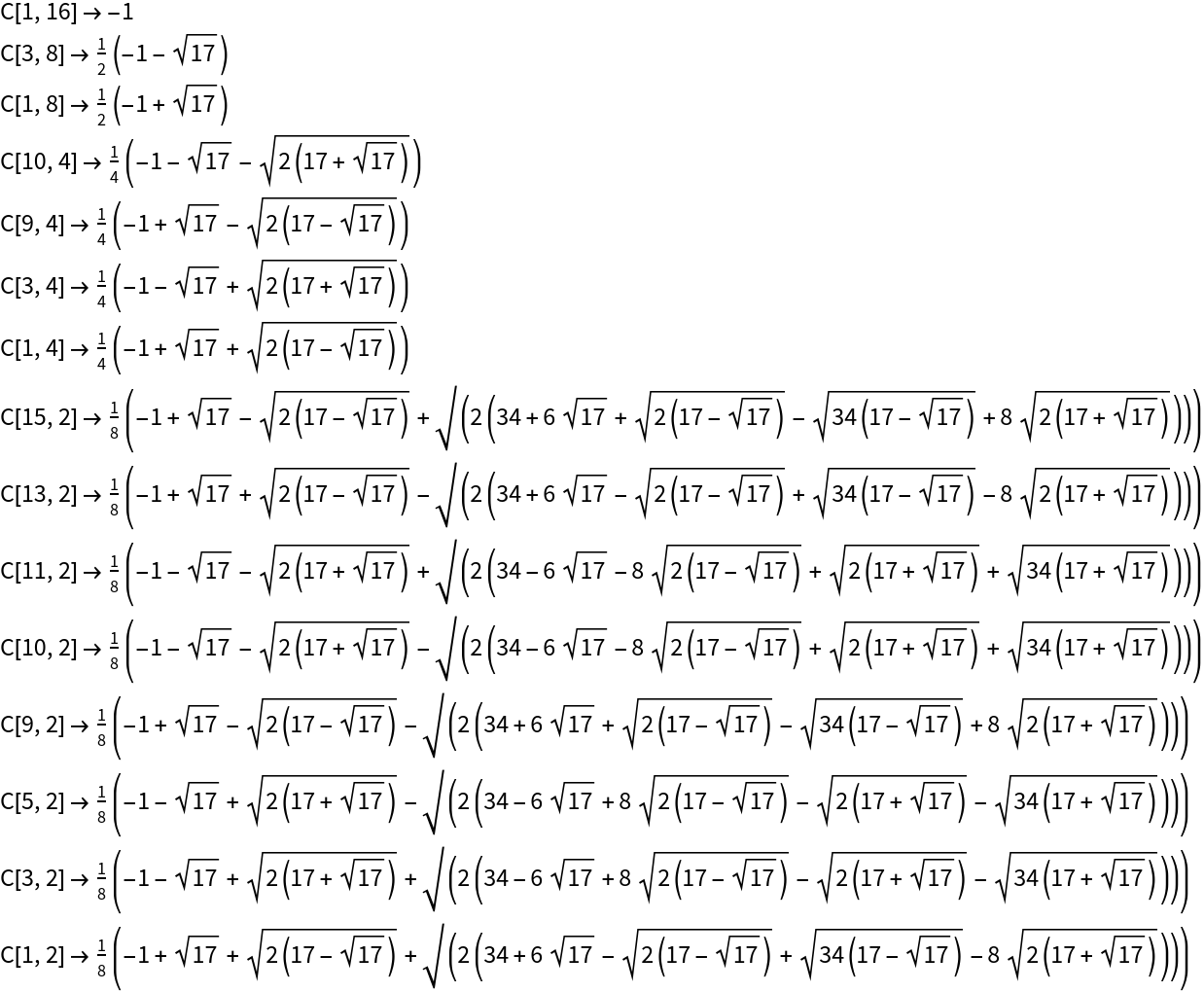### Properties and Relations

The function ToRadicals does express cos(2π/257) in radicals, but not in square roots:

 In:=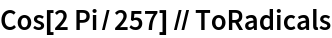Out=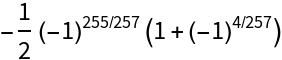ToRadicals of cos(2π/17) in returns an expression quite similar to Cos2PiOverFermatPrime:

 In:=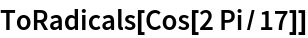Out=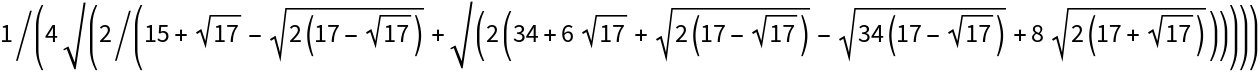In:=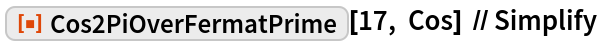Out=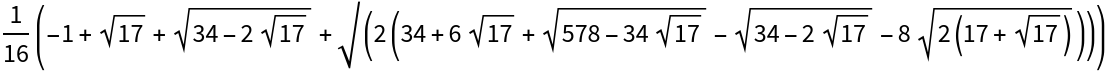In:=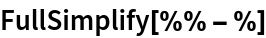Out=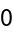### Possible Issues

The computation for the Fermat prime 65537 will take about 10 to 15 min on a modern laptop:

 In:=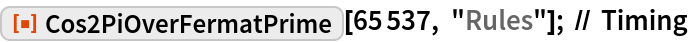Out=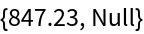### Neat Examples

The value of cos(2π/65537) was first computed by Johann Gustav Hermes and took him 10 years. The computation are still preserved in the famous “suitcase of Göttingen”:

 In:=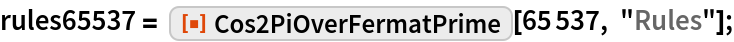The length of the rule list is p-2:

 In:=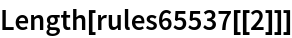Out=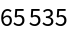To compute the period values, we change the rules to assignments and let the 65535 assignments autoevaluate after numericalization of numbers:

 In:=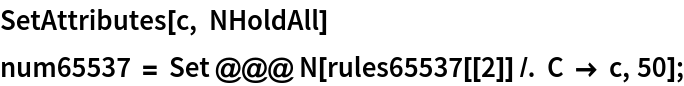The last value is just cos(2π/65537) :

 In:=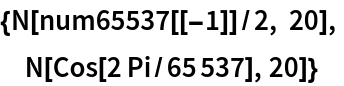Out=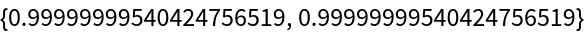Plot the distribution of the 65535 period values on a linear and on a logarithmic scale:

 In:=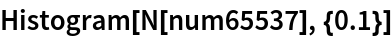Out=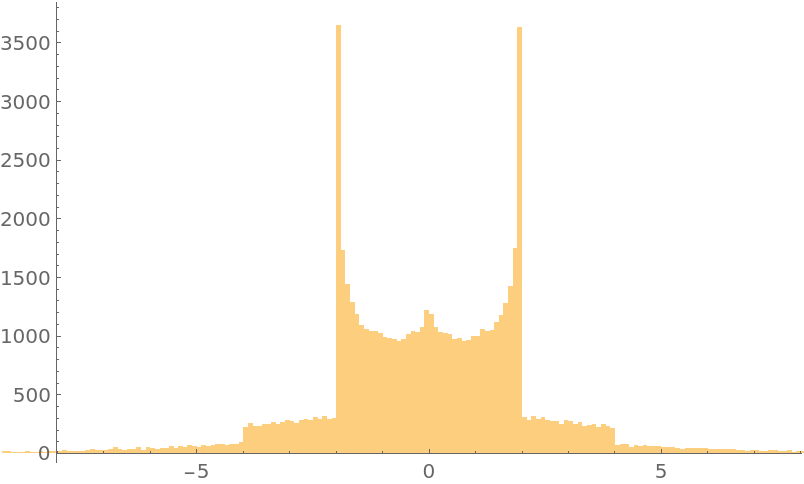In:=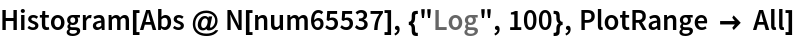Out=